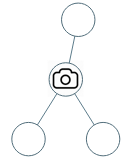## Algorithm

Problem Name: 968. Binary Tree Cameras

You are given the `root` of a binary tree. We install cameras on the tree nodes where each camera at a node can monitor its parent, itself, and its immediate children.

Return the minimum number of cameras needed to monitor all nodes of the tree.

Example 1:```Input: root = [0,0,null,0,0]
Output: 1
Explanation: One camera is enough to monitor all nodes if placed as shown.
```

Example 2:```Input: root = [0,0,null,0,null,0,null,null,0]
Output: 2
Explanation: At least two cameras are needed to monitor all nodes of the tree. The above image shows one of the valid configurations of camera placement.
```

Constraints:

• The number of nodes in the tree is in the range `[1, 1000]`.
• `Node.val == 0`

## Code Examples

### #1 Code Example with Java Programming

```Code - Java Programming```

``````
class Solution {
private final int NOT_MONITORED = 0;
private final int MONITORED_NOCAM = 1;
private final int MONITORED_WITHCAM = 2;

public int minCameraCover(TreeNode root) {
if (root == null) {
return 0;
}
int[] cameras = {0};
int top = dfs(root, cameras);
return cameras + (top == NOT_MONITORED ? 1 : 0);
}

private int dfs(TreeNode root, int[] cameras) {
if (root == null) {
return MONITORED_NOCAM;
}
int left = dfs(root.left, cameras);
int right = dfs(root.right, cameras);
if (left == MONITORED_NOCAM && right == MONITORED_NOCAM) {
return NOT_MONITORED;
} else if (left == NOT_MONITORED || right == NOT_MONITORED) {
cameras++;
return MONITORED_WITHCAM;
} else {
return MONITORED_NOCAM;
}
}
}
``````
Copy The Code &

Input

cmd
root = [0,0,null,0,0]

Output

cmd
1

### #2 Code Example with Javascript Programming

```Code - Javascript Programming```

``````
const minCameraCover = function(root) {
if (root === null) return 0;
let max = 0;
return (helper(root) < 1 ? 1 : 0) + max;
function helper(root) {
if (root === null) return 2;
if (root.left === null && root.right === null) return 0;
let left = helper(root.left);
let right = helper(root.right);
if (left === 0 || right === 0) {
max++;
return 1;
}
return left === 1 || right === 1 ? 2 : 0;
}
};
``````
Copy The Code &

Input

cmd
root = [0,0,null,0,0]

Output

cmd
1

### #3 Code Example with Python Programming

```Code - Python Programming```

``````
class Solution:
res = 0
def minCameraCover(self, root):
def dfs(root):
if not root: return 2
if not root.left and not root.right: return 0
l, r = dfs(root.left), dfs(root.right)
if l == 0 or r == 0:
self.res += 1
return 1
if l == 1 or r == 1:
return 2
return 0
return (dfs(root) == 0) + self.res
``````
Copy The Code &

Input

cmd
root = [0,0,null,0,null,0,null,null,0]

Output

cmd
2